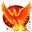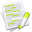#wx.lib.floatcanvas.FCObjects.PointsObjectMixin¶

A mixin class that provides some methods suitable for use with objects that have a set of (x, y) coordinate pairs.

##Class Hierarchy¶Inheritance diagram for class PointsObjectMixin:

##Methods Summary¶

 CalcBoundingBox Calculate the bounding box. Move Moves the object by delta, where delta is a (dx, dy) pair. SetPoints Sets the coordinates of the points of the object to Points (NX2 array).

##Class API¶

class PointsObjectMixin

A mixin class that provides some methods suitable for use with objects that have a set of (x, y) coordinate pairs.

### Methods¶

CalcBoundingBox(self)

Calculate the bounding box.

Move(self, Delta)

Moves the object by delta, where delta is a (dx, dy) pair.

Parameters

Delta – is a (dx, dy) pair ideally a NumPy array of shape (2, )

SetPoints(self, Points, copy=True)

Sets the coordinates of the points of the object to Points (NX2 array).

Parameters
• Points

takes a 2-tuple, or a (2,) NumPy array of point coordinates

• copy (boolean) – By default, a copy is made, if copy is set to False, a reference is used, if Points is a NumPy array of Floats. This allows you to change some or all of the points without making any copies.

For example:

Points = Object.Points
# shifts the points 5 in the x dir, and 10 in the y dir.
Points += (5, 10)
# Sets the points to the same array as it was
Object.SetPoints(Points, False)